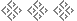The Meunch Formula
Danny Kleinman, 1991
But Only Hogs Win Backgammons, © 1991 Danny KleinmanDean Muench, one of the Chicago area’s strongest backgammon players, has contributed to the theory of the game for a long time. His computer simulations of long races in 1980 shook my faith in the cube recommendations then current (based simplistically on the ratio of the lead to the length of the race) and led me to develop Normal Race Takes and The Racer’s Edge.

More recently, Dean has sought shortcuts for estimating match equities. Some tournament players have mastered match charts by rote, of course. In the middle of a match, however, it is far better to be able to compute an estimate from a formula than to rely on a memorized chart.

The Meunch Approximation was one such attempt. Admittedly inaccurate, it was still better than nothing. Now Dean has outdone himself with the Meunch Formula, which supercedes the Meunch Approximation. The Meunch Formula is accurate to about one percentage point. Those of us who have tried to develop formulae for match equities can appreciate Dean’s remarkable achievement of accuracy.

The Meunch Formula can be stated in many ways, but before I give it, I should caution that it applies only prior to the Crawford game. That is, neither player may be within a point of winning the match.

The simplest way to use the Muench Formula begins with a conversion to the equivalent score in an 11-point match. For example, a 6–3 score in a 9-point match becomes 8–5. A 19–12 score in a 23-point match becomes 7–0. A 23–15 score in a 25-point match becomes 9–1.

What about scores like 10–8 in a 23-point match or 12–0 in a 15-point match which cannot be converted to equivalent 11-point match scores? The Muench Formula does not apply. It covers neither close scores in the early stages of a long match nor lopsided scores (where the lead exceeds 9 points) in any match.

One of the reasons I require conversion to an 11-point match is precisely to exclude scores for which the Meunch Formula produces inaccurate estimates. (Other ways of stating the Muench Formula would not result in the automatic exclusion of the inappropriate cases.)

Ready? All right. The formula we seek is for W, the probability that the leader will win the match, expressed in percentage terms. Let L be the leader’s score, T the trailer’s score, and D the difference (LT) between the two. Then

W = 50 +
 (L − T)(10 + T) 2
= 50 +
 D(10 + T) 2
.

Usually this gives answers expressed in whole percentages, but occasionally the answers are in half percents. If you are uncomfortable dealing with half percents, you will usually introduce only the smallest error by rounding the leader’s winning percentage to the next higher whole percent.

## Alternate Form

Dean insists that some who try to use this formula will forget to convert to the equivalent score in an 11-point match. So, at his suggestion, I give a version which doesn’t require conversion. Let M be the match length. Then

W = 50 +
 (L − T)(21 + T − M) 2
= 50 +
 D(21 + T − M) 2
.

At the cost of one extra variable, you are spared the work of conversion.The Muench Formula (using “away” scoring)
At the start of any game prior to the Crawford game:
• Let T be the number of points Trailer is away from winning the match.
• Let D be the difference in the players’ scores.
The percentage probability of Leader winning the match is roughly:
50 +
 D(21 − T) 2